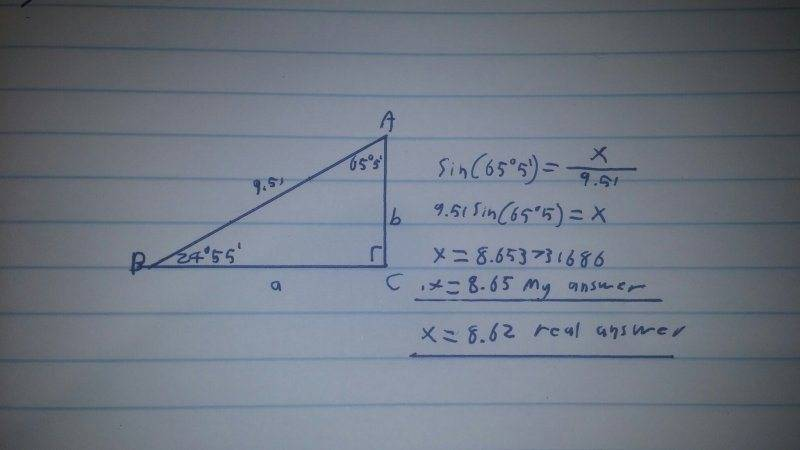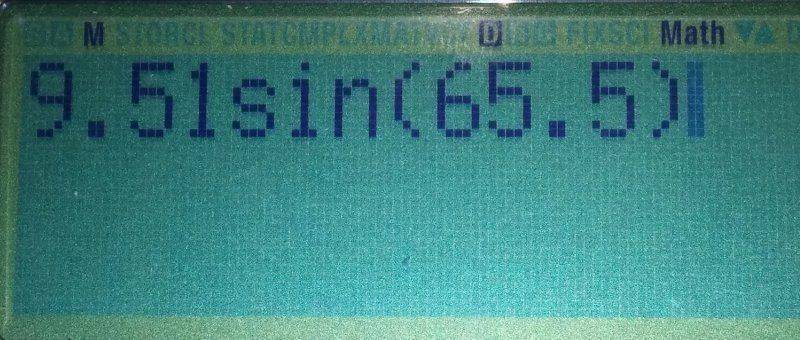• Tyler2358

#### Tyler2358

Hey Guys. I'm having a bit of a problem with my solving triangles book. I'm finding the book really easy but there's this one thing that I keep getting wrong. Whenever I'm working with degrees with decimal points my answer aways fluctuates slightly from the real answer. I must be doing something wrong. Any help will be greatly appreciated!I'm getting 8.62. Can you show me your input into your calculator? How are you expressing the minutes for example?

Actually, I see what you've done. You expressed $65^o5'$ as 65.5 in your calculator. This is not correct!

$$65.5^o=65^o30'$$

because $0.5^o$ is half of a degree, and that is equal to 30' since $60'=1^o$.

The opposite angle $24^o55'$ is NOT to be expressed as 24.55 in the calculator, but rather 24+55/60. Think about it in terms of time. The degree is the hour and the minute is the minute. What is 4 lots of 2 hours and 5 minutes equal to? Obviously it'll be 8 hours and 20 minutes. Let's check it though:

It's not 2.5*3 = 7.5, but rather 2 hours * 3 + 5/60 hours * 3 = 6 hours + 15/60 hours = 6 hours and 15 minutes.

This is what you want to put into your calculator:

$$\sin(65+5/60)*9.51$$

if your calculator is expressed in degrees.

I'm getting 8.62. Can you show me your input into your calculator? How are you expressing the minutes for example?
I did think maybe I'm calculating the minutes wrong but for the life of me I couldn't find anything on the internet about it.Oh I see now. Thank you for helping me! Finally I've been stuck here for a while now.

You're welcome!

Also keep in mind that your calculator might also have a degrees, minutes and seconds button. You can use this button to easily express the degrees and minutes with $65^o5^o$ on the calculator, which would be equivalent to $65^o5'=65+5/60$. Also, if you start using seconds too, then
$$65^o5^o20^o$$ on your calculator would be equivalent to $$65^o5'20''=65+5/60+20/60^2=65+5/60+20/3600$$

I did think maybe I'm calculating the minutes wrong but for the life of me I couldn't find anything on the internet about it.

It's always going to be in the last place you look:http://en.wikipedia.org/wiki/Degree_(angle)

Little details like that are what makes space probes fail to go into orbit around Mars.